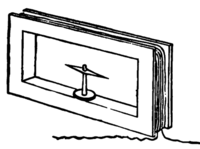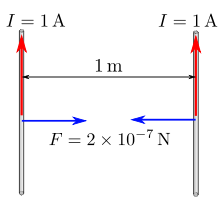# Ampere

﻿
AmpereCurrent can be measured by a galvanometer, via the deflection of a magnetic needle in the magnetic field created by the current.

The ampere (SI symbol: A; non-SI abbreviation: amp) is the SI unit of electric current (quantity symbol: I, i) and is one of the seven SI base units. It is named after André-Marie Ampère (1775–1836), French mathematician and physicist, considered the father of electrodynamics. Note that SI supports only the use of symbols and deprecates the use of any abbreviations for units.

In practical terms, the ampere is a measure of the amount of electric charge passing a point in an electric circuit per unit time with 6.241 × 1018 electrons, or one coulomb per second constituting one ampere.

The practical definition may lead to confusion with the definition of a coulomb (i.e., 1 amp-second), but in practical terms this means that measures of a constant current (e.g., the nominal flow of charge per second through a simple circuit) will be defined in amps (e.g., "a 20 mA circuit") and the flow of charge through a circuit over a period of time will be defined in coulombs (e.g., "a variable-current circuit that flows a total of 10 coulombs over 5 seconds"). In this way, amperes can be viewed as a "rate of flow" and coulombs viewed as an "amount of flow."

## DefinitionIllustration of the definiton of the ampere unit.

Ampère's force law states that there is an attractive or repulsive force between two parallel wires carrying an electric current. This force is used in the formal definition of the ampere, which states that it is "the constant current that will produce an attractive force of 2 × 10–7 newton per metre of length between two straight, parallel conductors of infinite length and negligible circular cross section placed one metre apart in a vacuum".

The SI unit of charge, the coulomb, "is the quantity of electricity carried in 1 second by a current of 1 ampere". Conversely, a current of one ampere is one coulomb of charge going past a given point per second:$\rm 1\ A=1\tfrac C s$

That is, in general, charge Q is determined by steady current I flowing for a time t as Q = It.

In practice, its name is often shortened to amp.

## History

The ampere was originally defined as one tenth of the CGS system electromagnetic unit of current (now known as the abampere), the amount of current that generates a force of two dynes per centimetre of length between two wires one centimetre apart. The size of the unit was chosen so that the units derived from it in the MKSA system would be conveniently sized.

The "international ampere" was an early realization of the ampere, defined as the current that would deposit 0.001118000 grams of silver per second from a silver nitrate solution. Later, more accurate measurements revealed that this current is 0.99985 A.

## Realization

The standard ampere is most accurately realized using a watt balance, but is in practice maintained via Ohm's Law from the units of electromotive force and resistance, the volt and the ohm, since the latter two can be tied to physical phenomena that are relatively easy to reproduce, the Josephson junction and the quantum Hall effect, respectively.

At present, techniques to establish the realization of an ampere have a relative uncertainty of approximately a few parts in 107, and involve realizations of the watt, the ohm and the volt.

## Proposed future definition

Rather than a definition in terms of the force between two current-carrying wires, it has been proposed to define the ampere in terms of the rate of flow of elementary charges. Since a coulomb is approximately equal to 6.2415093×1018 elementary charges, one ampere is approximately equivalent to 6.2415093×1018 elementary charges per second, such as electrons, moving past a boundary in one second. The proposed change would define 1 A as being the current in the direction of flow of a particular number of elementary charges per second. In 2005, the International Committee for Weights and Measures (CIPM) agreed to study the proposed change. The new definition is expected to be formally proposed at the 25th General Conference on Weights and Measures (CGPM) in 2015.

## Everyday examples

The current drawn by typical constant-voltage energy distribution systems is usually dictated by the power (watts) consumed by the system and the operating voltage. For this reason the examples given below are grouped by voltage level.

• Hearing aid (typically 1 mW at 1.4 V): 0.7 mA

### Motor vehicles – 12 V DC

A typical motor vehicle has a 12 V battery. The various accessories that are powered by the battery might include:

• Instrument panel light (typically 2 W): 166 mA.
• Headlights (typically 60 W): 5 A each.
• Starter Motor (typically 1–2 kW): 80-160 A

### US & Canada domestic supply – 120 V AC

Most United States domestic power suppliers run at 120 V.

Household circuit breakers typically provide a maximum of 15 A or 20 A of current to a given set of outlets.

• 22-inch/56-centimeter Portable Television (35 W): 290 mA
• Tungsten light bulb (60–100 W): 500–830 mA
• Toaster, kettle (2 kW): 16.6 A
• Immersion heater (4.6 kW): 38.3 A

### European domestic supply – 230 V AC

Most European domestic power supplies run at 230 V, so the current drawn for a particular appliance will be less than for an equivalent United States appliance. This means that lighter (and cheaper) cabling can be used.[citation needed]

The current drawn by a number of typical appliances are:

• 22-inch/56-centimeter Portable Television (35 W): 150 mA
• Tungsten light bulb (60–100 W): 250–450 mA
• Toaster, kettle (2 kW): 9 A
• Immersion heater (4.6 kW): 20 A

Wikimedia Foundation. 2010.

### Look at other dictionaries:

• ampère — [ ɑ̃pɛr ] n. m. • 1865; de Ampère, nom d un physicien ♦ Unité d intensité des courants électriques, qui correspond à un écoulement de un coulomb par seconde dans un conducteur (symb. A). Ampère par mètre (symb. A/m) :unité d intensité de champ… …   Encyclopédie Universelle

• AMPÈRE (A.-M.) — Dans l’effort continu de l’homme pour dominer la matière, capter son énergie, interpréter ses manifestations et les utiliser, des noms illustres émergent à qui nous sommes redevables de toute cette transformation. Dans la multitude de savants,… …   Encyclopédie Universelle

• Ampere — Ampère Cette page d’homonymie répertorie les différents sujets et articles partageant un même nom. Ampère peut désigner : André Marie Ampère (1775 1836), physicien français. Son nom a été donné à : l’ampère, unité SI d’intensité d’un… …   Wikipédia en Français

• Ampere —     André Marie Ampère     † Catholic Encyclopedia ► André Marie Ampère     Physicist and mathematician, b. 22 January, 1775, at Lyons, France; d. at Marseilles, 10 June, 1836. His father was a prosperous and educated merchant, his mother… …   Catholic encyclopedia

• Ampère — can refer to: *Ampère (car) *Ampere (unit) *André Marie Ampère, one of the main discoverers of electromagnetism *Ampere (band) …   Wikipedia

• Ampère — ampere (de «A. M. Ampère», físico francés) m. Fís. Amperio, en la nomenclatura internacional. * * * ampere. (De A. M. Ampère, 1775 1836, matemático y físico francés). m. Fís. amperio. * * * …   Enciclopedia Universal

• Ampère — (Париж,Франция) Категория отеля: 4 звездочный отель Адрес: 102 Avenue de Villiers, 17 й округ …   Каталог отелей

• Ampère — (spr. angpǟr ), 1) André Marie, Physiker und Mathematiker, geb. 22. Jan. 1775 in Lyon, gest. 10. Juni 1836 in Marseille, studierte Chemie und Physik, erhielt 1801 eine Professur in Bourg, ging von dort nach Lyon und 1805 nach Paris und ward 1809… …   Meyers Großes Konversations-Lexikon

• ampere — (de «A. M. Ampère», físico francés) m. Fís. Amperio, en la nomenclatura internacional. * * * ampere. (De A. M. Ampère, 1775 1836, matemático y físico francés). m. Fís. amperio. * * * ► masculino METR …   Enciclopedia Universal

• ampere — (n.) 1881, the current that one volt can send through one ohm, from Fr. ampère, named for French physicist André Marie Ampère (1775 1836). Shortened form amp is attested from 1886 …   Etymology dictionary

• Ampere — Am p[ e]re ([aum]N p[^a]r ), Ampere Am*pere ([a^]m*p[=a]r ), n. [From the name of a French electrician.] (Elec.) The unit of electric current; defined by the International Electrical Congress in 1893 and by U. S. Statute as, one tenth of the unit …   The Collaborative International Dictionary of English